# 最优化算法之粒子群算法（PSO）

PSO的优势：在于简单容易实现并且没有许多参数的调节。目前已被广泛应用于函数优化、神经网络训练、模糊系统控制以及其他遗传算法的应用领域。

1、基本思想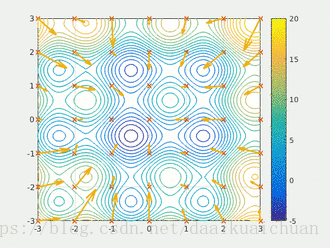2、更新规则

PSO初始化为一群随机粒子(随机解)。然后通过迭代找到最优解。在每一次的迭代中，粒子通过跟踪两个“极值”(pbest，gbest)来更新自己。在找到这两个最优值后，粒子通过下面的公式来更新自己的速度和位置。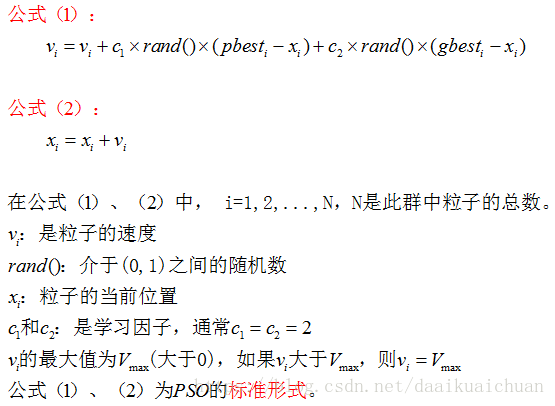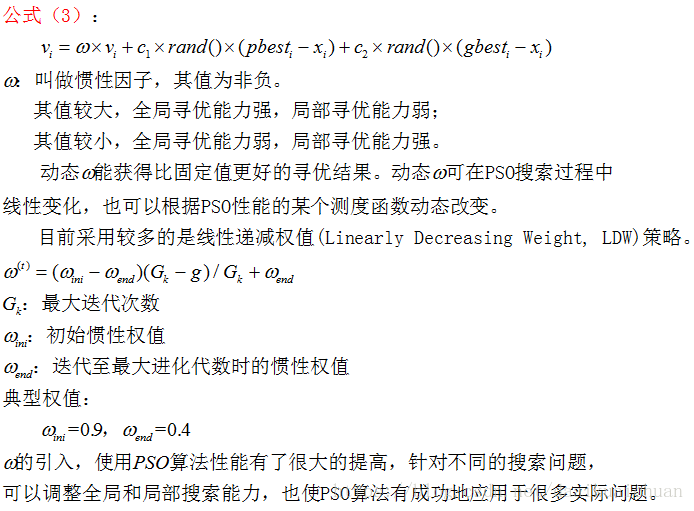3、PSO算法的流程和伪代码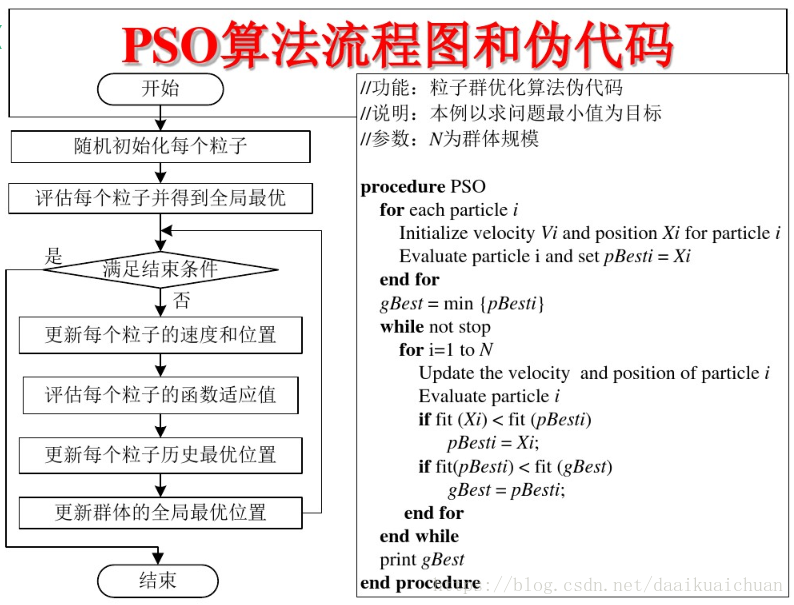4、PSO算法举例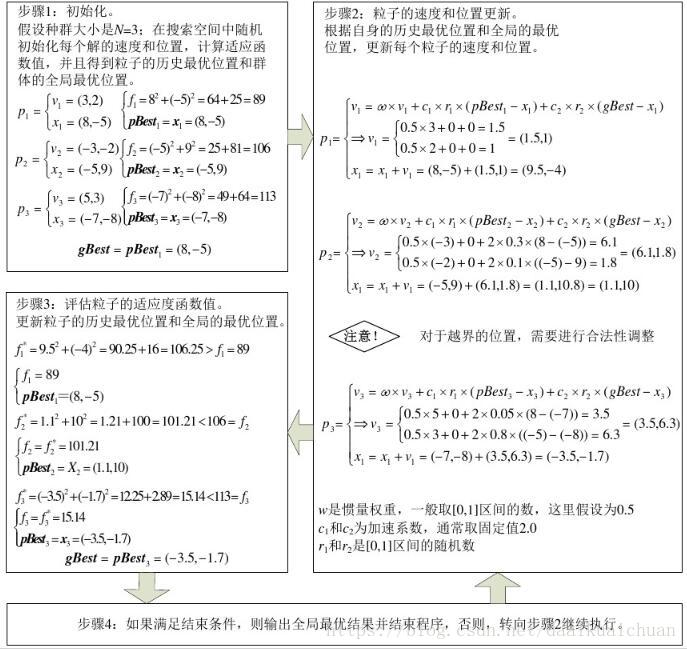5、PSO算法的demo

``````#include <iostream>
#include <vector>
#include <cmath>
#include <map>
#include <algorithm>
#include <random>
#include <ctime>
#include <Eigen/Dense>
using namespace Eigen;
using namespace std;

const int dim = 1;//维数
const int p_num = 10;//粒子数量
const int iters = 100;//迭代次数
const int inf = 999999;//极大值
const double pi = 3.1415;
//定义粒子的位置和速度的范围
const double v_max = 4;
const double v_min = -2;
const double pos_max = 2;
const double pos_min = -1;
//定义位置向量和速度向量
vector<double> pos;
vector<double> spd;
//定义粒子的历史最优位置和全局最优位置
vector<double> p_best;
double g_best;
//使用eigen库定义函数值矩阵和位置矩阵
Matrix<double, iters, p_num> f_test;
Matrix<double, iters, p_num> pos_mat;

//定义适应度函数
double fun_test(double x)
{
double res = x * x + 1;
return res;
}

//初始化粒子群的位置和速度
void init()
{
//矩阵中所有元素初始化为极大值
f_test.fill(inf);
pos_mat.fill(inf);
//生成范围随机数
static std::mt19937 rng;
static std::uniform_real_distribution<double> distribution1(-1, 2);
static std::uniform_real_distribution<double> distribution2(-2, 4);
for (int i = 0; i < p_num; ++i)
{
pos.push_back(distribution1(rng));
spd.push_back(distribution2(rng));
}
vector<double> vec;
for (int i = 0; i < p_num; ++i)
{
auto temp = fun_test(pos[i]);//计算函数值
//初始化函数值矩阵和位置矩阵
f_test(0, i) = temp;
pos_mat(0, i) = pos[i];
p_best.push_back(pos[i]);//初始化粒子的历史最优位置
}
std::ptrdiff_t minRow, minCol;
f_test.row(0).minCoeff(&minRow, &minCol);//返回函数值矩阵第一行中极小值对应的位置
g_best = pos_mat(minRow, minCol);//初始化全局最优位置
}

void PSO()
{
static std::mt19937 rng;
static std::uniform_real_distribution<double> distribution(0, 1);
for (int step = 1; step < iters; ++step)
{
for (int i = 0; i < p_num; ++i)
{
//更新速度向量和位置向量
spd[i] = 0.5 * spd[i] + 2 * distribution(rng) * (p_best[i] - pos[i]) +
2 * distribution(rng) * (g_best - pos[i]);
pos[i] = pos[i] + spd[i];
//如果越界则取边界值
if (spd[i] < -2 || spd[i] > 4)
spd[i] = 4;
if (pos[i] < -1 || pos[i] > 2)
pos[i] = -1;
//更新位置矩阵
pos_mat(step, i) = pos[i];
}
//更新函数值矩阵
for (int i = 0; i < p_num; ++i)
{
auto temp = fun_test(pos[i]);
f_test(step, i) = temp;
}
for (int i = 0; i < p_num; ++i)
{
MatrixXd temp_test;
temp_test = f_test.col(i);//取函数值矩阵的每一列
std::ptrdiff_t minRow, minCol;
temp_test.minCoeff(&minRow, &minCol);//获取每一列的极小值对应的位置
p_best[i] = pos_mat(minRow, i);//获取每一列的极小值，即每个粒子的历史最优位置
}
g_best = *min_element(p_best.begin(), p_best.end());//获取全局最优位置
}
cout << fun_test(g_best);
}

int main()
{
init();
PSO();
system("pause");
return 0;
}``````